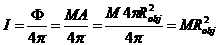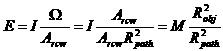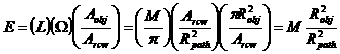## Monday, July 23, 2012

### Investigating Irradiance Error III: Estimated E

In the last two posts, we set out to find the relationship between four radiometric quantities:

• Exitance (M): the power density emitted by a surface, measured in Watts / m2. We will reserve B for the per-micron un-integrated quantity.
• Radiance (L): the power density emitted by a surface into each solid angle, measured in Watts / m2 / steradian.
• Intensity (I): the power emitted by a point source into each solid angle, measured in Watts / steradian.
• Irradiance (E): the power incident upon a receiving surface, measured in Watts / m2.

We discovered that the relationship between exitance and radiance followed the simple formula for a lambertian surface:  M = πL.  We will now look at the relationship between these and  irradiance.  If anything, properly calculating this last is the most critical; as its name implies, radiometry is about using the power incident on our measuring device to infer the power being emitted from the surface of interest.

Let us assume that a spherical object of radius Robj is sufficiently far away from our sensor that we can treat it as a point source of intensity I.  The total power Φ it emits is equal to the product of its exitance M and surface area A; its intensity I is that power divided by the total surrounding solid angle, 4π.  Thus:You will remember that intensity is in units of Watts / steradian.  Thus, if we assume that the sensor is looking directly at the emitting object, we can find the irradiance at the aperture of our receiver by multiplying the intensity by the solid angle Ω subtended by the aperture and dividing by the area Arcvr of the aperture.  Remembering that the definition of solid angle is the ratio of the area of a sphere subtended by that angle divided by the square of the radius gives us:where Rpath is the distance from the emitting object to our sensor.

Alternatively, we can treat our emitting object as a disk of radius Robj.  It’s radiance L is the exitance M divided by π as found previously.  The irradiance on a sensor at distance  is the product of the radiance, the solid angle Ω subtended by the sensor’s aperture, and the disk area Aobj = πRobj2, divided by the area of the sensor aperture:Thus, we can see that whether we approximate our emitting object of radius Robj as a point source or a disk, we get the same result for the amount of radiation incident on a sensor at distance Rpath.  (The textbook irradiance of, for instance, the sun at the top of the earth’s atmosphere is 1480W/m2, for instance.)

However, we must point out the obvious:  an emitting sphere is not a disk, and it may not appear as a point source.  For instance, the sun’s dimensions are apparent to an observer on the earth, 93M miles away.

In our next post, we will calculate the exact irradiance on the aperture of a sensor from a spherical emitting object.

(Note:  I apologize for the crappy looking equations.  I have a procedure for cleaning them up, but it’s tedious, and I’m tired.)